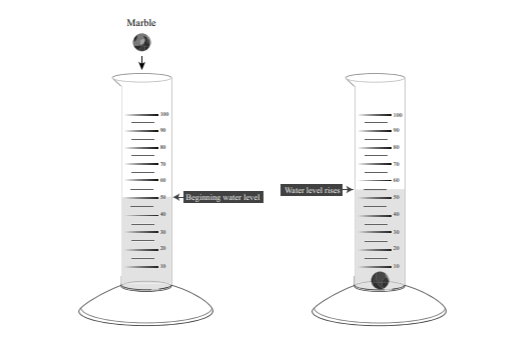Scan QR code or get instant email to install app

Question:

# An experiment was conducted to determine the density of a marble. First, the student massed the marble with a triple beam balance to determine it had a mass of 10 grams. Second, the student filled the graduated cylinder with water as shown in the diagram and then dropped in the marble. Using the diagram, calculate the density of the marble.A 2 g/ml.
explanation

10 g/5 ml = 2 g/ml. The formula for density is mass divided by volume. The mass of the marble is 10 grams, and the volume of the displaced water is 5 milliliters.